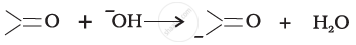# PUC Science Class 11 - Karnataka Board PUC Question Bank Solutions

Subjects
Topics
Subjects
Popular subjects
Topics

< prev  1 to 20 of 5824  next >

Pyruvate undergoes oxidative decarboxylation to produce _____________.

(a) 2-PGA

(b) α-Ketoglutarate

(c) Succinyl-Co-A

(d) Acetyl-Co-A

[0.14] Respiration in Plants
Chapter: [0.14] Respiration in Plants
Concept: Phases of Respiration: Glycolysis

After a long deep breath, we do not respire for some seconds due to ______.

[0.17] Breating and Exchange of Gases (Part B)
Chapter: [0.17] Breating and Exchange of Gases (Part B)
Concept: Breathing – Respiratory Cycle

Give the diagrammatic representation of ETS

[0.14] Respiration in Plants
Chapter: [0.14] Respiration in Plants
Concept: Phases of Respiration: Oxidative Phosphorylation

In a standard ECG which one of the following alphabets is the correct representation of the respective of the human heart ______.

[0.18] Body Fluids and Circulation (Part A)
Chapter: [0.18] Body Fluids and Circulation (Part A)
Concept: Circulatory Pathways > Electrocardiograph (ECG)

Sketch and label the ‘Ultrastructure of Chloroplast’.

[0.13] Photosynthesis in Higher Plants
Chapter: [0.13] Photosynthesis in Higher Plants
Concept: Site of Photosynthesis

What are the reactions involved for ozone layer depletion in the stratosphere?

[0.14] Environmental Chemistry
Chapter: [0.14] Environmental Chemistry
Concept: Atmospheric Pollution > Stratospheric Pollution

The reaction, $\ce{CO(g) + 3H2(g) ↔ CH4(g) + H2O(g)}$ is at equilibrium at 1300 K in a 1L flask. It also contains 0.30 mol of CO, 0.10 mol of H2 and 0.02 mol of H2O and an unknown amount of CH4 in the flask. Determine the concentration of CH4 in the mixture. The equilibrium constant, Kc for the reaction at the given temperature is 3.90.

[0.07] Equilibrium
Chapter: [0.07] Equilibrium
Concept: Law of Chemical Equilibrium and Equilibrium Constant

For the following bond cleavages, use curved arrows to show the electron flow and classify homolysis or heterolysis. Identify reactive intermediate produced as free radical, carbocation and carbanion.

$\ce{CH3O - OCH3 -> CH3\overset\bullet{\text{O}} + \overset\bullet{\text{O}}CH3}$

[0.12] Organic Chemistry - Some Basic Principles and Techniques
Chapter: [0.12] Organic Chemistry - Some Basic Principles and Techniques
Concept: Fundamental Concepts in Organic Reaction Mechanism > Fission of a Covalent Bond

For the following bond cleavages, use curved-arrows to show the electron flow and classify as homolysis or heterolysis. Identify reactive intermediate produced as free radical, carbocation and carbanion.[0.12] Organic Chemistry - Some Basic Principles and Techniques
Chapter: [0.12] Organic Chemistry - Some Basic Principles and Techniques
Concept: Fundamental Concepts in Organic Reaction Mechanism > Fission of a Covalent Bond

Draw the resonance structure for the following compound. Show the electron shift using curved-arrow notation.

$\ce{CH3CH = CH\overset{+}{C}H2}$

[0.12] Organic Chemistry - Some Basic Principles and Techniques
Chapter: [0.12] Organic Chemistry - Some Basic Principles and Techniques
Concept: Fundamental Concepts in Organic Reaction Mechanism > Resonance Structure

Why do elements in the same group have similar physical and chemical properties?

[0.03] Classification of Elements and Periodicity in Properties
Chapter: [0.03] Classification of Elements and Periodicity in Properties
Concept: Periodic Trends in Chemical Properties > Periodicity of Valence or Oxidation States

Suppose, the electron in a hydrogen atom makes transition from n = 3 to n = 2 in 10−8 s. The order of the torque acting on the electron in this period, using the relation between torque and angular momentum as discussed in the chapter on rotational mechanics is

[0.02] Structure of Atom
Chapter: [0.02] Structure of Atom
Concept: Bohr’s Model for Hydrogen Atom

Find the degree measure corresponding to the following radian measure:
$\frac{9\pi}{5}$

[0.01] Trigonometric Functions
Chapter: [0.01] Trigonometric Functions
Concept: Concept of Angle

Find the degree measure corresponding to the following radian measure:
$- \frac{5\pi}{6}$

[0.01] Trigonometric Functions
Chapter: [0.01] Trigonometric Functions
Concept: Concept of Angle

Find the degree measure corresponding to the following radian measure:
$\left( \frac{18\pi}{5} \right)$

[0.01] Trigonometric Functions
Chapter: [0.01] Trigonometric Functions
Concept: Concept of Angle

Find the degree measure corresponding to the following radian measure:
(−3)c

[0.01] Trigonometric Functions
Chapter: [0.01] Trigonometric Functions
Concept: Concept of Angle

Find the degree measure corresponding to the following radian measure:
11c

[0.01] Trigonometric Functions
Chapter: [0.01] Trigonometric Functions
Concept: Concept of Angle

Find the degree measure corresponding to the following radian measure:
1c

[0.01] Trigonometric Functions
Chapter: [0.01] Trigonometric Functions
Concept: Concept of Angle

Find the radian measure corresponding to the following degree measure:
300°

[0.01] Trigonometric Functions
Chapter: [0.01] Trigonometric Functions
Concept: Concept of Angle

Find the radian measure corresponding to the following degree measure: 35°

[0.01] Trigonometric Functions
Chapter: [0.01] Trigonometric Functions
Concept: Concept of Angle
< prev  1 to 20 of 5824  next >